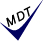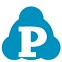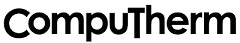## 熱力学データベース　PanTitanium```
ソフトウェア Pandat と用いることにより、
チタン合金の平衡状態図を計算できます。
(2016: Mn 追加)
(2020: W  追加) , (2022: Hf  追加)

チタン合金用（２１元素）           (2022)
Ti, Al, B, C, Cr, Cu, Fe, H, Hf, Mn, Mo, N, Nb, Ni, O, Si, Sn, Ta, V, W, Zr

PanTitanium
Developed for commercial Ti-rich alloys such as alpha, alpha+beta, and beta alloys.

More than 340 phases.

Composition range (in wt%) for application:
Al: less than 35
B : less than 0.5
C : less than 0.5
Cr: less than 5
Cu: less than 3
Fe: less than 3
H : less than 0.5
Hf: less than 0.5
Mn: less than 0.5
Mo: less than 8
N : less than 0.5
Nb: less than 8
Ni: less than 3
O : less than 0.5
Si: less than 0.5
Sn: less than 5
Ta: less than 8
Ti: balance (should be greater than 50)
V : less than 11
W : less than 8
Zr: less than 8

計算結果例

開発元：米国```